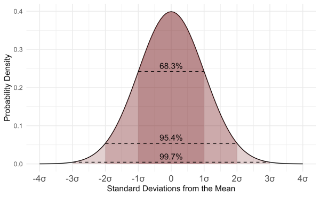# Statistical hypothesis testing

Topic | v1 | created by janarez |
Description

A statistical hypothesis is a hypothesis that is testable on the basis of observed data modelled as the realised values taken by a collection of random variables. A set of data is modelled as being realised values of a collection of random variables having a joint probability distribution in some set of possible joint distributions. The hypothesis being tested is exactly that set of possible probability distributions. A statistical hypothesis test is a method of statistical inference. An alternative hypothesis is proposed for the probability distribution of the data, either explicitly or only informally. The comparison of the two models is deemed statistically significant if, according to a threshold probability—the significance level—the data would be unlikely to occur if the null hypothesis were true.

##### Relations

In statistical hypothesis testing, a result has statistical significance when it is very unlikely to...Statistics is the discipline that concerns the collection, organization, analysis, interpretation, an...